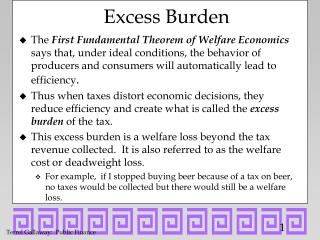DownloadDownload PresentationExcess Burden

# Excess Burden

Download Presentation## Excess Burden

- - - - - - - - - - - - - - - - - - - - - - - - - - - E N D - - - - - - - - - - - - - - - - - - - - - - - - - - -
##### Presentation Transcript

1. Excess Burden • The First Fundamental Theorem of Welfare Economics says that, under ideal conditions, the behavior of producers and consumers will automatically lead to efficiency. • Thus when taxes distort economic decisions, they reduce efficiency and create what is called the excess burden of the tax. • This excess burden is a welfare loss beyond the tax revenue collected. It is also referred to as the welfare cost or deadweight loss. • For example, if I stopped buying beer because of a tax on beer, no taxes would be collected but there would still be a welfare loss.

2. Initially, there are no economic distortions. Original budget constraint has a slope -(Px/Py) After a tax on x less x can be bought. The new budget constraint has a slope -[(1 + t)Px/Py]. Tax Shifts Budget Constraint y x

3. For any level of x, the vertical distance between the two budget constraints shows the tax payment measured in terms of the good y. Set Py = \$1 or let y represent all other goods. For example, if we hold purchases of x fixed at x1 then purchases of y would have to fall from y1 to y2 . Measuring the Tax Payment y y1 y2 x1 x

4. The tax causes the utility-maximizing bundle of goods to switch from 1 to 2. Naturally, utility decreases. The tax revenue collected is equal to the distance between A & B. Is the decrease in utility greater than the tax revenue collected? 2 B 1 A Decline in Utility y A x

5. Equivalent variation is the amount , if there were no tax, by which income would have to be reduced to yield an equivalent decline in utility. Such a reduction income is shown as a parallel shift of the budget constraint and is equal to the vertical distance between A and C. The distance between B & C is the dead weight loss (DWL). DWL is the difference between what is paid in taxes and an equivalent (in terms of utility) reduction in income y 2 B 1 C Equivalent Variation A x

6. A lump sum tax is a tax that is paid regardless of a consumer’s behavior. Significantly it doesn’t change relative prices. Rather, it simply decreases income by the amount of the tax. Note that reduction in income is identical to the tax collected. (The distance between A& B.) There is no Deadweight Loss. y A 2 1 B Lump Sum Tax x

7. Why no Lump Sum Taxes? • An example of such a tax is a head tax. • Such taxes are regressive. • If everybody has to pay \$100, this is much more of a burden to a poor person than it is to a billionaire. • Margaret Thatcher replaced property taxes with a head tax and was subsequently booted out of office.

8. Wherefore Excess Burden? • Remember, allocative efficiency requires that MRSxy = MRTxy • However, after the tax, the consumer faces a new budget constraint such that utility is maximized where MRSxy = (1 + tx)Px/Py. • For producers, the important thing is price net of taxes. Thus, profit-maximizing producers will still set MRTxy = Px/Py. • Since tx > 0, MRSxy > MRTxy. That is, the necessary condition for allocative efficiency is not satisfied.

9. Intuitive Explanation • As long as consumers are willing to cover the economic costs of producing a good, then economic efficiency dictates that the good should be produced. • However when a tax, rather than production costs, pushes a good beyond a consumer’s price range, then too few resources will be dedicated to the production of a good for which consumers would have been willing to pay. • Basically economists want decisions to reflect opportunity costs rather than the tax structure.

10. Before and after the tax on x, the consumption of x is x1. The excess burden is equal to BC. Equivalent Variation equals E1S Tax Revenues equal E1E2. E2S is the excess burden A lump sum tax resulting in the same reduction in utility would raise more revenue. (IC runs tangent to both new BCs) Tax did change consumption of Y & alter the relative mix of X & Y! Does Quantity Have to Change for There to be Inefficiency?

11. The move from our initial equilibrium (1) to our new equilibrium (2) can be divided into two parts: The move from 1 to 3 is the income effect. The move from 3 to 2 is the substitution effect. y 1 A B 2 C 3 D x1 x Substitution and Income Effects

12. Excess burden is caused when a tax changes relative prices and the consumer’s MRS--this is exactly what the substitution effect is. The income effect can offset or mask the substitution effect. While there is no perceived change in quantity demanded, there is still a deadweight loss. y 1 A B 2 C 3 D x1 x SE causes DWL • s